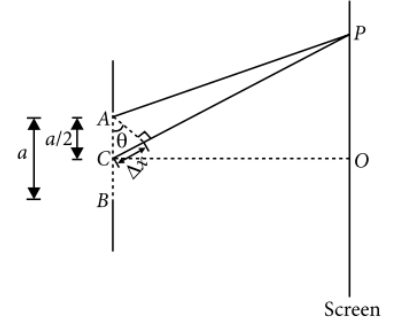Diffraction
Question

# At the first minimum adjacent to the central maximum of a single-slit diffraction pattern, the phase difference between the Huygen's wavelet from the edge of the slit and the wavelet from the midpoint of the slit is

Difficult
Solution

## The situation is shown in the figure.In figure A and B represent the edges of the slit AB of width a and C represents the midpoint of the slit. For the first minimum at P,For the first minimum at P,$\Delta x=\frac{a}{2}\mathrm{sin}\theta =\frac{1}{2}\left(a\mathrm{sin}\theta \right)$$\Delta \varphi =\frac{2\pi }{\lambda }\Delta x=\frac{2\pi }{\lambda }×\frac{\lambda }{2}=\pi$

Get Instant Solutions